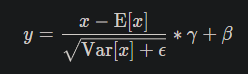# Why 2D batch normalisation is used in features and 1D in classifiers?

What is the difference between BatchNorm2d and BatchNorm1d? Why a BatchNorm2d is used in features and BatchNorm1d is used in classifier?

Hi,

There is no mathematical difference between them, except the dimension of input data.
nn.BatchNorm2d only accepts 4D inputs while nn.BatchNorm1d accepts 2D or 3D inputs. And because of that, in `features` which has been constructed of `nn.Conv2d` layers, inputs are `[batch, ch, h, w]` (4D) we need `BatchNorm2d` and in `classifier` we have `Linear` layers which accept `[batch, length]` or `[batch, channel, length]` (2D/3D) so we need `BatchNorm1d`.
Two linked docs completely explain this idea.

Bests

3 Likes

If there is no difference between them, then why would there be two different functions? And why wouldn’t there be a universal BatchNorm class that accepts inputs with arbitrary dimensions?

Hi

There is a universal BatchNorm!
Simply put here is the architecture (torch.nn.modules.batchnorm — PyTorch 1.11.0 documentation):

1. a base class for normalization, either `Instance` or `Batch` normalization → `class _NormBase(Module)`. This class includes no computation and does not implement `def _check_input_dim(self, input)`
2. Now we have `class _BatchNorm(_NormBase)` that extends `_NormBase` which actually does the computation and tracks the values necessary for it. In the last line, you see that class calls `return F.batch_norm(..)` in its `forward` function. We will talk about `F.batch_norm()` in a bit.
3. In the end, you have classes in form `class BatchNormXd(_BatchNorm)` that extend `_BatchNorm` and the only thing they do is to implement `_check_input_dim(self, input)` that was intentionally left behind in `_NormBase` class (see step 1).

About `torch.nn.functional.batch_norm` (from step 2): This function, does all the computation given values, in other words, if you want to mix all parts into a single class, you should be able too, but it would break the modularity, etc.

Here is an example that you can exactly replicate what `BatchNormXd` does for just a single forward pass given the normalization formulation:``````# Test case
x = torch.randn(2, 7)  # batch=2, features=7
running_mean = x.mean(dim=0)  # assuming 'mean' tracked during training
# Remark: as the documentation says, we must use biased estimator, i.e. 'unbiased=False'.
running_var = x.var(dim=0, unbiased=False)  # assuming 'var' tracked during training
gamma = None  # assuming 'gamma' is not set
beta = None  # assuming 'beta' is not set

>>> x
tensor([[ 1.6080,  1.5907, -1.0321,  1.0416, -0.8388,  0.0759, -0.9885],
[-0.1404,  0.7668,  1.4246, -0.4341, -1.0590,  0.7760,  0.8207]])

# BatchNorm as a function
import torch.nn.functional as F

>>> F.batch_norm(x, running_mean, running_var, gamma, beta, momentum=0.)
tensor([[ 1.0000,  1.0000, -1.0000,  1.0000,  0.9996, -1.0000, -1.0000],
[-1.0000, -1.0000,  1.0000, -1.0000, -0.9996,  1.0000,  1.0000]])

# BatchNorm as a class
import torch.nn as nn

bn1d = nn.BatchNorm1d(x.shape, affine=False, momentum=None)  # 'affine=False' sets beta and gamma to None
# you can verify mean and var by bn1d.running_mean and bn1d.running_var
# you can verify gamma and beta by bn1d.weight and bn1d.bias

>>> bn1d(x)
tensor([[ 1.0000,  1.0000, -1.0000,  1.0000,  0.9996, -1.0000, -1.0000],
[-1.0000, -1.0000,  1.0000, -1.0000, -0.9996,  1.0000,  1.0000]])
``````

Bests

1 Like

that’s very good, thanks for the pointer!